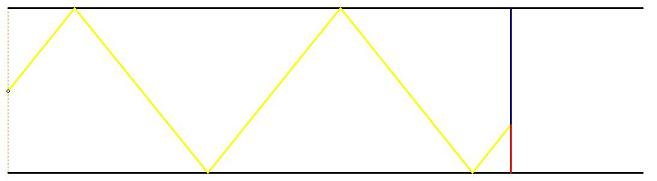#### You may also like### Diophantine N-tuples

Can you explain why a sequence of operations always gives you perfect squares?### DOTS Division

Take any pair of two digit numbers x=ab and y=cd where, without loss of generality, ab > cd . Form two 4 digit numbers r=abcd and s=cdab and calculate: {r^2 - s^2} /{x^2 - y^2}.### Sixational

The nth term of a sequence is given by the formula n^3 + 11n . Find the first four terms of the sequence given by this formula and the first term of the sequence which is bigger than one million. Prove that all terms of the sequence are divisible by 6.

# Screen Shot

##### Age 14 to 16Challenge Level
A moveable screen slides along a mirrored corridor towards a centrally placed light source. A ray of light from that source is directed towards a wall of the corridor, which it strikes at $45^\circ$ before being reflected across to the opposite wall and so on until it hits the screen.

If the screen is $20$ metres down the corridor from the light source and if the corridor is $2$ metres wide, find the position on the screen where the point of light appears.Part Two: Now remember that the screen is moveable. The distance, $d$, of the screen down the corridor can change, so the position where the point of light appears on the screen will depend on $d$. Can you find a function, expressing the position of the light on the screen in terms of $d$?

Part Three: If the ray leaves the source making an angle $\theta$ with the direction of the corridor, and the distance, $d$, of the screen down the corridor can still change, the position where the point of light appears on the screen will depend on $d$ and on $\theta$ . Can you find this function?